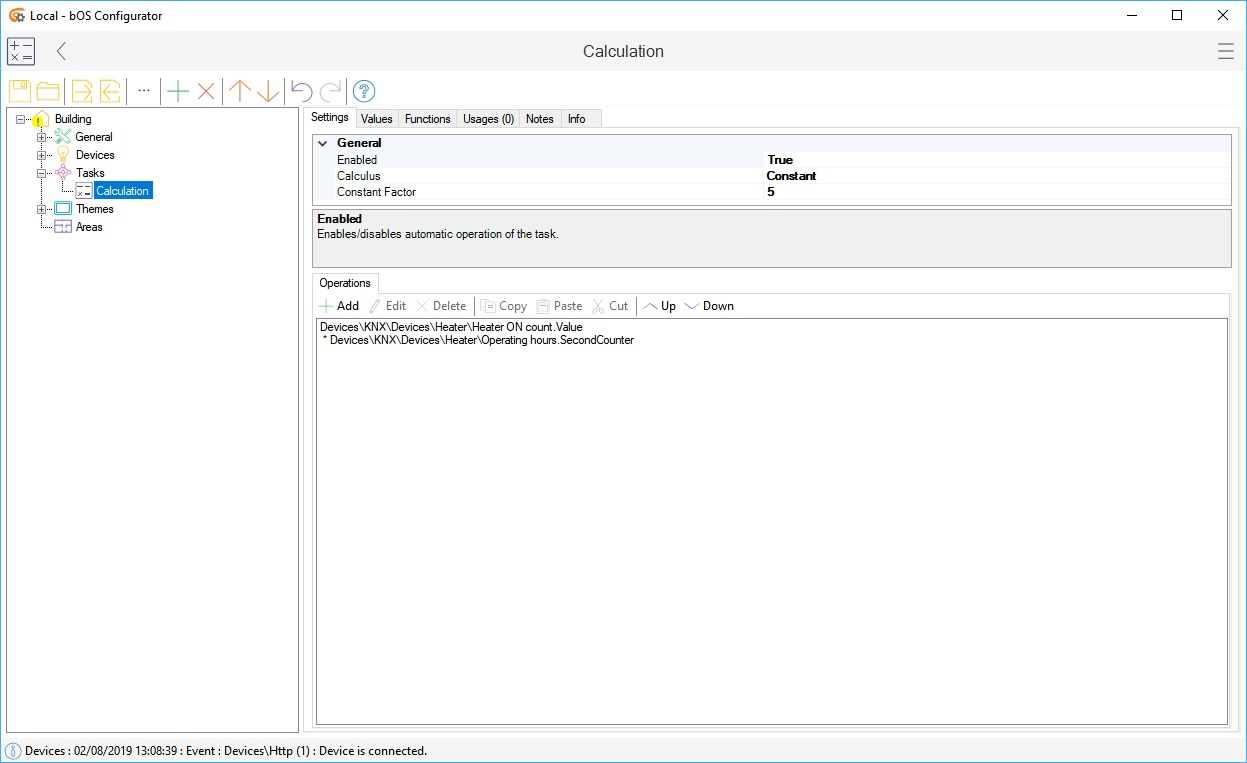# Calculation

Calculation performs mathematical operations. Result can be used for further building automation.

## Calculation

Calculation performs mathematical operations in bOS. Results can be used for further building automation.

Calculation task performs calculation of polynomial values. Polynome values can be object values or constants. The whole polynomial is multiplied with Constant Factor. Integration and differentiation Calculus can be applied to the polynome. Calculation is performed each time any of the input value is updated. Polynomial operations are set using the Operations list editor.

For each operation, the Operation Type can be selected (Addition, Subtraction, Multiplication, Division). Operation can contain only the Constant Factor or an Input Value (linked to an object value) scaled with the Constant Factor.

The result of the calculation is represented by Value. Int Value is Value converted into integer. Calculate function can be called to manually trigger the calculation task. Reset function resets integration and differentiation calculus and sets Value to 0.Picture 1: Calculation node

## Examples

### Example 1: Calculate wind speed in km/h from input value measured in m/s.

1m/s = 3.6km/h.

1. Set Constant Factor to: 3.6.

### Example 2: Calculate energy (kWh) from current input value measured in amperes (A).

Energy is calculated as integration of power:

E(kWh) = Int(U*I)dt * 1/3600 / 1000

1. Set Calculus to: Integral.

2. Voltage (U) can be set as a constant (e.g. 230V). In this case Constant Factor is: 230 * 1/3600 / 1000 = 6,3e-5.

4. Use Value output in tasks or make a Double user interface control for it. A Run Function control linked to the Reset function can be used to enable resetting of the counter value.

### Example 3: Count the number of ON switching

1. Create a counter variable (Devices->Variables->Integer).